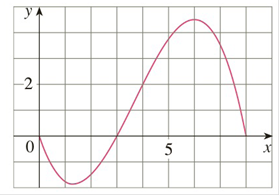Chapter 4.2, Problem 51E

Chapter
Section
Textbook Problem

# For the function / whose graph is shown, list the following quantities in increasing order, from smallest to largest, and explain your reasoning.(A) ∫ 0 8 f ( x ) d x (B) ∫ 0 3 f ( x ) d x (C) ∫ 3 8 f ( x ) d x (D) ∫ 4 8 f ( x ) d x (E) f ′ ( 1 )To determine

To list:

The given quantities in theincreasing order from the smallest to the largest and explainthe reasoning.

Explanation

1) Concept:

A definite integral can be interpreted as a net area, that is, as difference of areas;

abf(x)dx=A1-A2

where, A1 is the region above x- axis and below the graph of  f, and A2 is the region below x- axis and above the graph of f.

2) Given:

Graph:

3) Calculation:

First, calculate the area for different intervals of a function.

To calculate the area, count the squares between the curve and the x- axis within the considered interval

A) For the interval [0, 8]08fxdx03fxdx+3

### Still sussing out bartleby?

Check out a sample textbook solution.

See a sample solution

#### The Solution to Your Study Problems

Bartleby provides explanations to thousands of textbook problems written by our experts, many with advanced degrees!

Get Started

#### Find more solutions based on key concepts